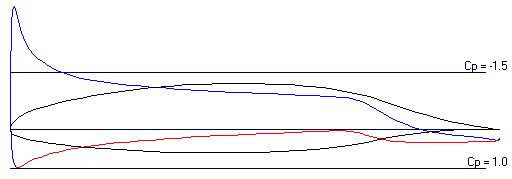## Pressure CoefficientThe pressure coefficient is defined as:

Cp = 1- [Surface Velocity / Free Stream Velocity] 2

Pressure Coefficient vs Surface

Blue represents regions of low pressure (velocity greater than the free stream conditions) and red represents high pressure (velocity less than the free stream conditions). Green represents a pressure coefficient of 0.0.

Calculated values along the surface may be examined by scrolling the cursor while holding down the left mouse button.## Pressure Coefficient vs Chord

Blue represents the Pressure Coefficient along the upper surface and red along the lower surface. If the viscous option has been selected, the approximate transition points are shown.

The transition from laminar to turbulent flow is shown by the first blue or red circle. The point of separation (if any) is shown by the next blue or red circle.The displayed results as a function of the angle of attack are a linear interpolation of the calculated values.

The Display Scale slider may be used to increase or decrease the relative size of the display.

Calculated values along the surface may be examined by scrolling the cursor while holding down the left mouse button. A vertical line will show the selected chord location.